# Unbalanced data (SMOTE)

## Intro

Imbalanced data typically refers to a problem with classification problems where the classes are not represented equally.For example, you may have a 2-class (binary) classification problem with 100 instances (rows). A total of 80 instances are labeled with Class-1 and the remaining 20 instances are labeled with Class-2. This is an imbalanced dataset and the ratio of Class-1 to Class-2 instances is 80:20 or more concisely 4:1. You can have a class imbalance problem on two-class classification problems as well as multi-class classification problems. Most techniques can be used on either.

Most classification data sets do not have exactly equal number of instances in each class, but a small difference often does not matter.

There are problems where a class imbalance is not just common, it is expected. For example, in datasets like those that characterize fraudulent transactions are imbalanced. The vast majority of the transactions will be in the “Not-Fraud” class and a very small minority will be in the “Fraud” class. Another example is customer churn datasets, where the vast majority of customers stay with the service (the “No-Churn” class) and a small minority cancel their subscription (the “Churn” class). When there is a modest class imbalance like 4:1 in the example above it can cause problems.

The accuracy paradox is the name for the exact situation in the introduction to this post. It is the case where your accuracy measures tell the story that you have excellent accuracy (such as 90%), but the accuracy is only reflecting the underlying class distribution. It is very common, because classification accuracy is often the first measure we use when evaluating models on our classification problems.

This is a short excusrion on the SMOTE (learn more about SMOTE, see the original 2002 paper titled “SMOTE: Synthetic Minority Over-sampling Technique“) variations I found and which allow to manipulate in various ways the creation of synthetic samples.

You can find the code of this exploration here, note that it’s Python v3.5.

import sys
import sklearn.datasets

from unbalanced_dataset import SMOTE
from sklearn import tree
from sklearn.ensemble import RandomForestClassifier
from sklearn import decomposition
import time, os
import pandas as pd, numpy as np
from ggplot import *
import matplotlib.pyplot as plt
from sklearn.metrics import confusion_matrix, roc_auc_score
%matplotlib inline

import sklearn.datasets
import matplotlib.pyplot as plt
import seaborn as sns
import datetime
from sklearn.decomposition import PCA
import pandas as pd, numpy as np, os, time

sns.set()

def plotClassificationData(x, y, title=""):
palette = sns.color_palette()
plt.scatter(x[y == 0, 0], x[y == 0, 1], label="Class #0", alpha=0.5,
facecolor=palette, linewidth=0.15)
plt.scatter(x[y == 1, 0], x[y == 1, 1], label="Class #1", alpha=0.5,
facecolor=palette, linewidth=0.15)
plt.title(title)
plt.legend()
plt.show()

def linePlot(x, title=""):
palette = sns.color_palette()
plt.plot(x, alpha=0.5, label=title, linewidth=0.2)
plt.legend()
plt.show()

def savePlotClassificationData(x, y):
palette = sns.color_palette()
plt.scatter(x[y == 0, 0], x[y == 0, 1], label="Class #0", alpha=0.5,
facecolor=palette, linewidth=0.15)
plt.scatter(x[y == 1, 0], x[y == 1, 1], label="Class #1", alpha=0.5,
facecolor=palette, linewidth=0.15)

plt.legend()
# plt.show()
filePath = "/Users/Swa/Desktop/" + str(datetime.datetime.now(datetime.timezone.utc).timestamp()) + ".png"
plt.savefig(filePath)

def plotHistogram(x, bins=10):
plt.hist(x, bins=bins)
plt.show()


Let’s create some classification data and we take 200 informative features. The usage of PCA to turn it into a 2D dataset is simply a projection technique so things can be plotted.

x,y = sklearn.datasets.make_classification(n_classes=2, class_sep=2, weights=[0.1, 0.9],
n_informative=200, n_redundant=0, flip_y=0,
n_features=200, n_clusters_per_class=1,
n_samples=5000, random_state=10)
# we'll invert the y to reflect the situation where '1' means classified
y = 1 - y

def count_classifieds(z): return sum(z)
def count_unclassifieds(z): return len(z) - sum(z)
def imbalance_ratio(z): return round(count_classifieds(z)/count_unclassifieds(z),1)
num_classified = count_classifieds(y)
num_unclassified = count_unclassifieds(y)
print("Number of classified clients: %s"%num_classified)
print("Number of unclassified clients: %s"%num_unclassified )
print("Imbalance ratio: %s"%imbalance_ratio(y))
pca = decomposition.PCA(n_components=2)
xv = pca.fit_transform(x)
plotClassificationData(xv,y)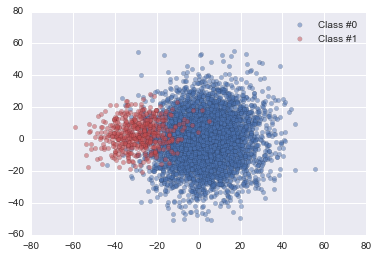In order to measure how the synthetic samples influence the classification we will use a random forest and naive Bayes classifiers.

def makeForest(X:np.array, Y:np.array, treecount=10):
if treecount <= 1: raise ValueError("The forest should not have less than one tree.")
model = RandomForestClassifier(n_estimators=treecount)
return model.fit(X, Y)

def getForestArea(x, y, treecount=10):
forest = makeForest(x, y, treecount)
predicted = forest.predict(x)
try:
area = roc_auc_score(y, predicted)
except Exception as exc:
area = 0
return round(area,2)

So, in the default setup without any SMOTE we have
print("Baseline AUC area: %s"%getForestArea(x,y))
Baseline AUC area: 0.92

## Standard SMOTE algorithm

The idea of the algorithm is to take k-nearest neighbors which define through the barycenter a direction and use a random factor in this direction. The larger the value of k the more the synthetic sample blur the existing ones. By default the value is 5.

Let’s first consider how the amount/ratio influence the accuracy of the predictions.

areas = []
division = np.arange(0.2,4,0.1)
for k in division:
smote = SMOTE(kind="regular", ratio=k)
sx, sy = smote.fit_transform(x, y)
areas.append(getForestArea(sx,sy))
plt.plot(division, areas)
plt.xlabel('Ratio vs. area.')
plt.ylabel('Area')
plt.title('AUC')
plt.ylim([0.97,1.005])
plt.show()
print("Ended with %s classified and %s unclassifieds."%(count_classifieds(sy),count_unclassifieds(sy)))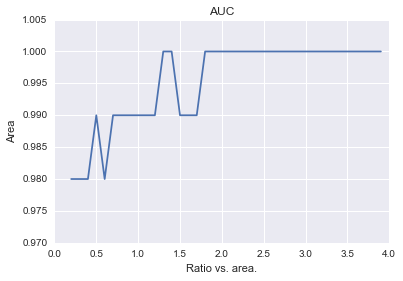Ended with 2450.0 classified and 2550.0 unclassifieds.

No surprise here, the more samples the more the accuracy increases. We end up with an almost 1:1 ratio.

Let’s assume now a fixed ration but increase the amount of nearest neighbors.

areas = []
division = np.arange(2,20,1)
for nn in division:
smote = SMOTE(kind="regular", k=nn, ratio=0.5)
sx, sy = smote.fit_transform(x, y)
areas.append(getForestArea(sx,sy))
plt.plot(division, areas)
plt.xlabel('Neighbors vs. area.')
plt.ylabel('Area')
plt.title('AUC')
plt.ylim([0.93,1.005])
plt.show()
print("At each turn we had %s classified and %s unclassifieds."%(count_classifieds(sy),count_unclassifieds(sy)))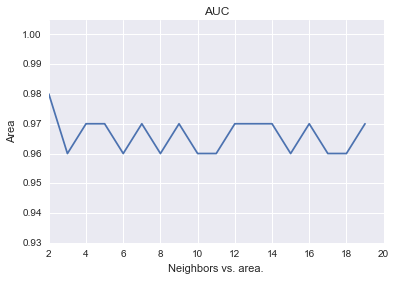At each turn we had 750.0 classified and 4250.0 unclassifieds.

So, the amount of neighbors diminishes the accuracy somewhat but there is no clear effect.

## Borderline 1 variation

The core idea of SMOTE is to use nearest neighbors to create new samples. However, if a minority point is close to another class then that point should rather not be considered since it would pull towards more noise and a less clear distinction between classes. So, the basic premise of the borderline SMOTE method is to identify points which potentially increase the confusion and not include these in the vectors creating new samples.
It’s clear that this method will have no effect if the classes are well separated and mostly effective when mixture is moderate.

areas = []
division = np.arange(0.2,4,0.1)
for k in division:
smote = SMOTE(kind="borderline1", ratio=k)
sx, sy = smote.fit_transform(x, y)
areas.append(getForestArea(sx,sy))
plt.plot(division, areas)
plt.xlabel('Ratio vs. area.')
plt.ylabel('Area')
plt.title('AUC')
plt.ylim([0.97,1.005])
plt.show()
print("Ended with %s classified and %s unclassifieds."%(count_classifieds(sy),count_unclassifieds(sy)))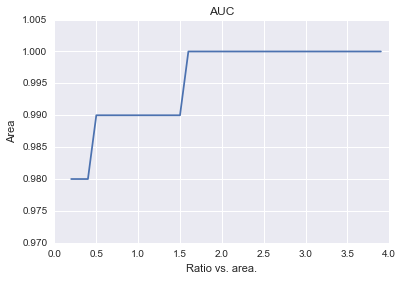Ended with 2450.0 classified and 2550.0 unclassifieds.


We can see that the accuracy goes more directly to its max due to the fact that our sample has indeed some noisy overlap between the clases and that the borderline SMOTE is really ideal in this case.

## SVM variation

This approach is similar to the borderline idea but one uses a support vector machine to detect boundary points and separate them for the creation of synthetic samples.

import warnings
warnings.filterwarnings('ignore')
areas = []
division = np.arange(0.2,4,0.1)
for k in division:
smote = SMOTE(kind="svm", ratio=k)
sx, sy = smote.fit_transform(x, y)
areas.append(getForestArea(sx,sy))
plt.plot(division, areas)
plt.xlabel('Ratio vs. area.')
plt.ylabel('Area')
plt.title('AUC')
plt.ylim([0.97,1.005])
plt.show()
print("Ended with %s classified and %s unclassifieds."%(count_classifieds(sy),count_unclassifieds(sy)))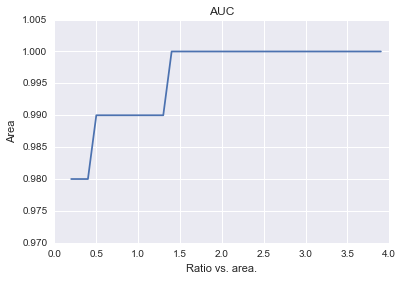Ended with 2449.0 classified and 2551.0 unclassifieds.

This gives a slightly better speed towards maximization (around 1.4 vs 1.6 with the borderline approach) but at the cost of a more lengthy computing time.
Tags: